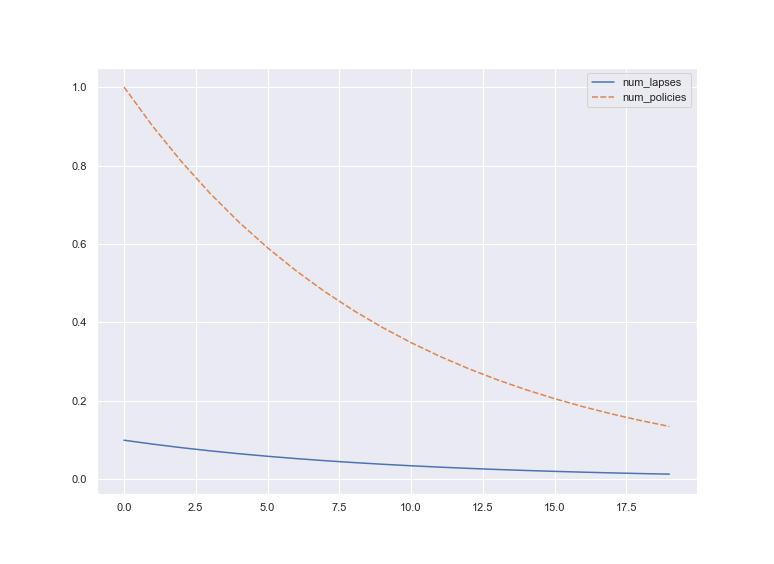## Model Walkthrough

This section breaks down the individual segments of code within the example model outlined in the index.

The overall model is:

``````import pandas as pd
import seaborn as sns
sns.set()

from heavymodel import Model

class PersistencyModel(Model):
def num_policies(self, t):
if t == 0:
return 1
else:
return self.num_policies(t-1) - self.num_lapses(t-1)

def num_lapses(self, t):
return 0.1 * self.num_policies(t)

model = PersistencyModel()

model._run(20)

df = pd.DataFrame({"num_lapses":model.num_lapses.values, "num_policies":model.num_policies.values})
sns.lineplot(data=df)
``````

### Import libraries

We first import required libraries.

``````import pandas as pd
import seaborn as sns
sns.set()

from heavymodel import Model
``````

`pandas` and `seaborn` are popular data and plotting libraries, for displaying the results. `from heavymodel import Model` makes the core `Model` class available.

### Define the class

The user model is defined as a class, as follows:

``````class PersistencyModel(Model):
def num_policies(self, t):
if t == 0:
return 1
else:
return self.num_policies(t-1) - self.num_lapses(t-1)

def num_lapses(self, t):
return 0.1 * self.num_policies(t)
``````

To make use of the functionality of `heavymodel.Model`, the user model inherits from this, as a sub-class.

Functions (`num_policies`, `num_lapses`) are defined as methods in the class. Each of these returns a result depending on the value of `t`.

Python methods take `self` as the first parameter, this refers to the instance of the class, when called, the `self` parameter is omitted, such as `self.num_policies(t-1)`. If a data item (attribute) such as `age` was added, the value of it could be accessed through `self.age`.

For more information on python classes, see docs.python.org/3/tutorial/classes.html or other reputable tutorials.

## Run the model

``````model = PersistencyModel()

model._run(20)
``````

`model = PersistencyModel()` creates an instance of the model, with all the methods bound to it. For example, `model.num_policies(50)` would evaluate `num_policies` at `t=50` (and all dependencies) and return the result. This allows models to be evaluated using the interactive prompt / REPL.

`model._run(20)` evaluates all the functions in the model from `t=0` to `t=19` (i.e. 20 time periods). Results are stored in `model.<method_name>.values`, e.g. `model.num_lapses.values`.

## Extract and plot results

``````df = pd.DataFrame({"num_lapses":model.num_lapses.values, "num_policies":model.num_policies.values})
sns.lineplot(data=df)
``````

This converts the results into a pandas dataframe, with column headers `num_lapses` and `num_policies`, and plots the result: